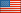# Policy, Data, Oversight Pay & Leave

## Fact Sheet: How to Compute FLSA Overtime Pay

### Background

Overtime pay for nonexempt employees is computed under the Fair Labor Standards Act (FLSA), subject to some special rules for Federal employees. Under the FLSA, overtime pay is determined by multiplying the employee's "straight time rate of pay" by all overtime hours worked PLUS one-half of the employee's "hourly regular rate of pay" times all overtime hours worked. All overtime work that is ordered or approved must be compensated. All overtime work that is "suffered or permitted" also must be compensated, except for flexible schedule employees. (See 5 CFR part 551.)

Include any applicable special rate supplement or locality payment in the "total remuneration" and "straight time rate of pay" when computing overtime pay under the FLSA. Compute the "hourly regular rate of pay" by dividing the "total remuneration" paid to an employee in the workweek by the number of hours in the workweek for which such compensation is paid.

### Pay Limitations

The limitation on an hourly rate of overtime pay under title 5, United States Code, does not apply to overtime pay under the FLSA. Also, the maximum biweekly or annual earnings limitations on title 5 premium pay do not apply to FLSA overtime pay.

### Computation

Multiply the straight time rate of pay by all overtime hours worked PLUS one-half of the employee's hourly regular rate of pay times all overtime hours worked. (See 5 CFR part 551, subpart E.)

### Example

Follow the steps below to compute FLSA overtime pay. The example below is based on a GS 7, step 1, annual rate of basic pay of \$43,251.  (See Salary Table 2020-RUS.)

Total Hours of Work: 52 hours.
Overtime Work: 12 hours.
Nightwork: 40 hours.
Sunday Work: 8 hours.

1. Hourly Rate of Basic Pay (Straight Time Rate of Pay).

\$43,251 / 2,087 hours = \$20.72.

2. Total Remuneration.

Basic Pay (40 hours).
\$20.72 x 40 hours = \$828.80.

Night Pay (40 hours).
\$20.72 x .10 = \$2.07.
\$2.07 x 40 hours = \$82.80.

\$20.72 x .25 = \$5.18.
\$5.18 x 8 hours = \$41.44.

Straight Time Pay.
\$20.72 x 12 hours = \$248.64.

Total Remuneration = \$ 1,201.68 (\$828.80 + \$82.80 + \$41.44 + \$248.64).

3. Hourly Regular Rate of Pay.

\$1,201.68 / 52 hours = \$23.11.

4. FLSA Overtime Pay.

Straight Time Rate of Pay x All Overtime Hours Worked.
\$20.72 x 12 hours = \$248.64.

One-half x Hourly Regular Rate of Pay x All Overtime Hours Worked.
0.5 x \$23.11 = \$11.56.
\$11.56 x 12 hours = \$138.72.

Total FLSA Overtime Pay = \$387.36 (\$248.64+ \$138.72).

5. Weekly Pay.

Basic Pay =\$828.80.
Night Pay = \$82.80.